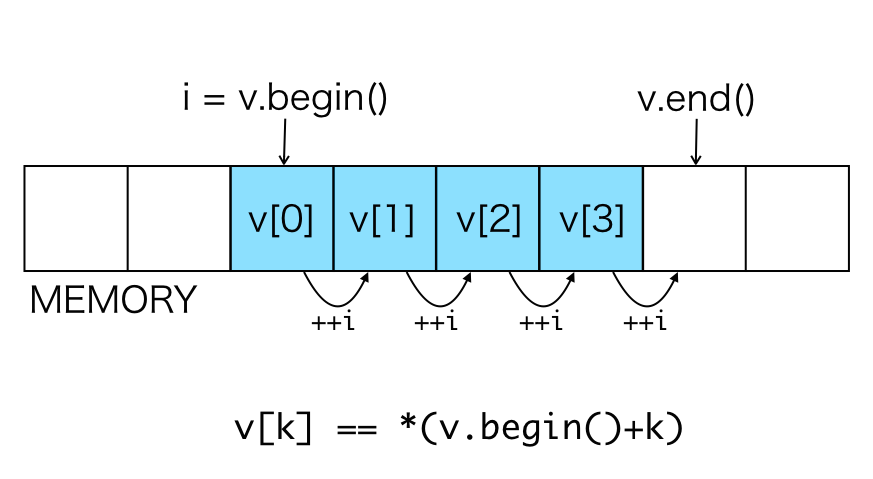# Chapter 28 Iterator

Iterator is an class used to access elements of `Vector` `DataFrame` `List`. If you want to use algorithms provided by standard C++, you need to understand iterator. Because many of the algorithms provided by standard C++ use iterators to specify location or range of data to apply the algorithms.

Specific iterator type is defined for each data structure of Rcpp.

``````NumericVector::iterator
IntegerVector::iterator
LogicalVector::iterator
CharacterVector::iterator
DataFrame::iterator
List::iterator``````

The figure below shows schematically how to access vector elements using an iterator.• `i = v.begin()` : The iterator `i` points to the first element of`v`.
• `++i` : Updates `i` to the state pointing to the next element.
• `--i` : Updates `i` to the state pointing to the previous element.
• `i + 1` : Represents an iterator pointing to the element 1 elements behind of `i`.
• `i - 1` : Represents an iterator pointing to the element 1 elements ahead of `i`.
• `*i` : Represents the value of the element pointed by `i`.
• `v.end()` : Represents an iterator pointing to the end (one after the last element) of `v`.
• `*(v.begin()+k)` : Represents the value of the `k`-th element of `v` (`v[k]`).

The following code example shows an example of traversing all the elements of a `NumericVector` using iterator to calculate sum of the elements.

``````// [[Rcpp::export]]
double rcpp_sum(NumericVector x) {
double total = 0;
for(NumericVector::iterator i = x.begin(); i != x.end(); ++i) {
total += *i;
}